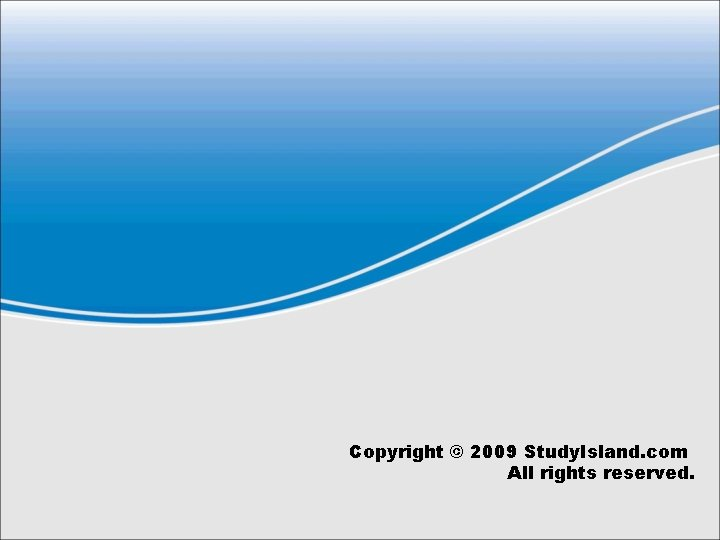# Triangles and Quadrilaterals Triangles have the following characteristics

• Slides: 11Triangles have the following characteristics: They are polygons. They have three sides. They have three angles. Their three angles total to 180°. They can be classified by their sides. They can be classified by their angles. The sum of the length of any two sides is greater than the third side.Classifying Triangles by their Sides equilateral isosceles scalene All 3 sides have the same length. At least two sides have the same length. No sides have the same length. Classify the following triangle by its sides: 5. 5 cm 3 cm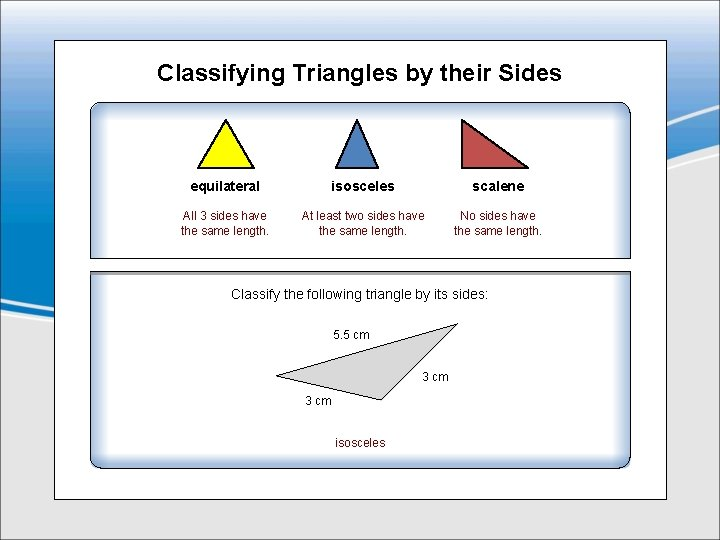Classifying Triangles by their Sides equilateral isosceles scalene All 3 sides have the same length. At least two sides have the same length. No sides have the same length. Classify the following triangle by its sides: 5. 5 cm 3 cm isosceles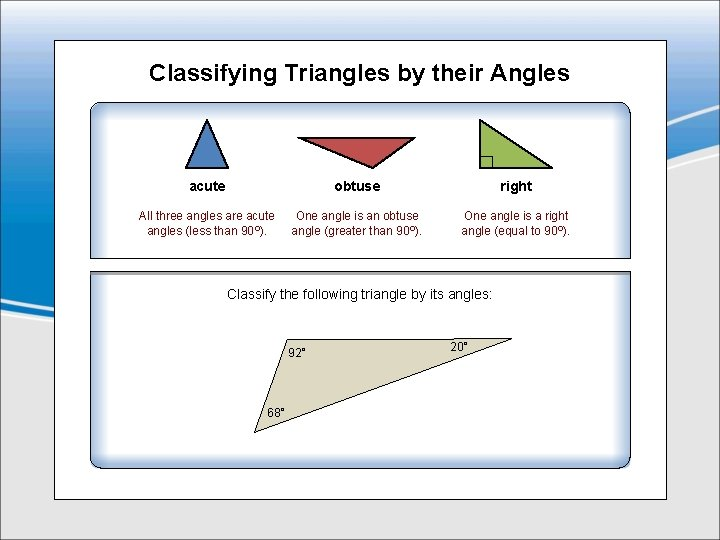Classifying Triangles by their Angles acute obtuse right All three angles are acute angles (less than 90°). One angle is an obtuse angle (greater than 90°). One angle is a right angle (equal to 90°). Classify the following triangle by its angles: 92° 68° 20°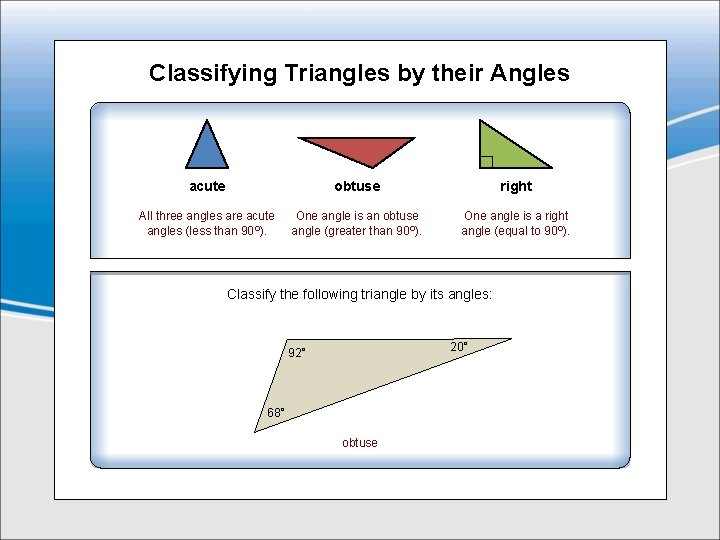Classifying Triangles by their Angles acute obtuse right All three angles are acute angles (less than 90°). One angle is an obtuse angle (greater than 90°). One angle is a right angle (equal to 90°). Classify the following triangle by its angles: 20° 92° 68° obtuse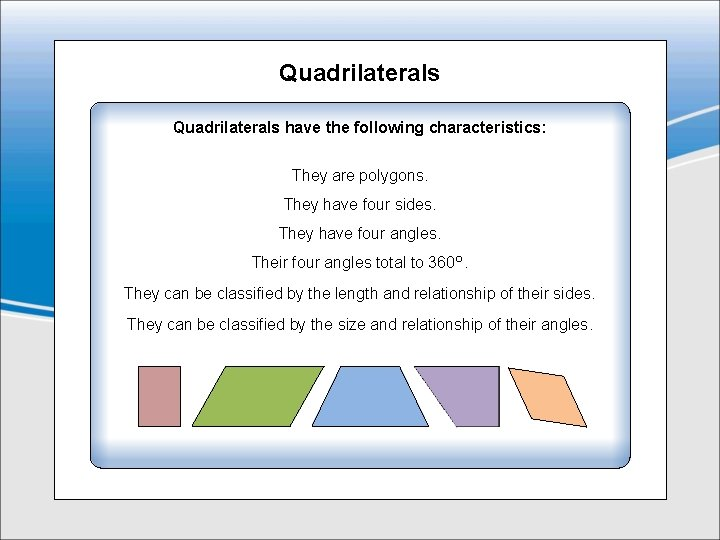Quadrilaterals have the following characteristics: They are polygons. They have four sides. They have four angles. Their four angles total to 360°. They can be classified by the length and relationship of their sides. They can be classified by the size and relationship of their angles.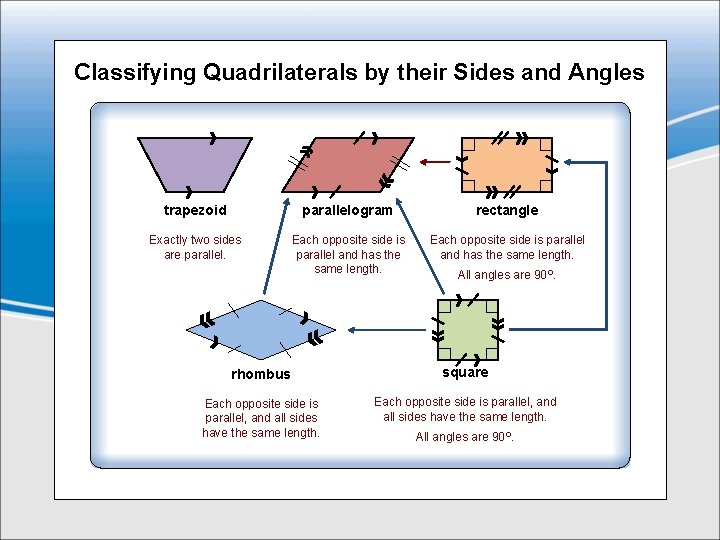Classifying Quadrilaterals by their Sides and Angles trapezoid parallelogram rectangle Exactly two sides are parallel. Each opposite side is parallel and has the same length. All angles are 90°. rhombus square Each opposite side is parallel, and all sides have the same length. All angles are 90°.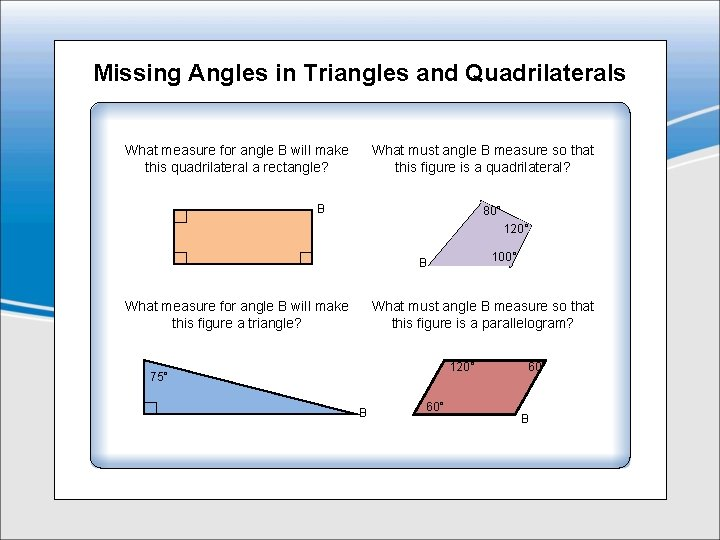Missing Angles in Triangles and Quadrilaterals What measure for angle B will make this quadrilateral a rectangle? What must angle B measure so that this figure is a quadrilateral? B 80° 120° 100° B What measure for angle B will make this figure a triangle? What must angle B measure so that this figure is a parallelogram? 120° 75° B 60° BMissing Angles in Triangles and Quadrilaterals What measure for angle B will make this quadrilateral a rectangle? What must angle B measure so that this figure is a quadrilateral? 90° 60° B 80° 120° 100° B What measure for angle B will make this figure a triangle? What must angle B measure so that this figure is a parallelogram? 15° 120° 75° B 60° B